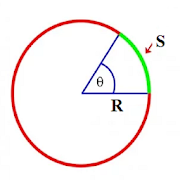# Arc CalculatorsEveryone
This app is build to Calculate followings:
Arc Length
Arc Angle to
Arc Cord Length
Arc Tangent height
Arc Depth
Arc Sector Area
Arc Segment Area

This Tool is useful in fabrication Filed.

Arc Length Calculator - In this app This Tool calculate arc length by using arc radius and arc angle.

Arc Radius Calculator - This tool is calculate arc radius by using arc angle and arc length.

Arc Angle calculator - This Tool is calculate arc angle by using arc radius and arc length.

Arc Depth Calculator - This tool Calculate Arc Depth by using radius and angle

Arc Tangent Height Calculator - This tool Calculate Arc Tangent Height Calculator by using Arc Radius and Arc Angle.

Arc Sector Area Calculator - This Tool Calculate Arc Sector area by using arc Angle and arc Radius

Arc Segment Area Calculator - This Tool calculate Arc Segment Area by Using Arc Angle and Radius

In this app Arc Angle and Arc Radius is used as input.

angle is used in Degree for Calculation.

This app is Very useful in fabrication Field. it required to calculate all arc calculation when we are doing Marking or we had required to calculate dimension while studying Engineering Drawings.

It is very helpful in Process Equipment fabrication, Piping, Engineering Drawings, Pressure Vessels fabrication, Heat Ex-changer Fabrication, Machining Process.
Collapse

## Reviews

Review policy and info

## What's New

Changes in App Layout.
Changes in App Theme.
Fix Minor Bugs.
Improve Performance.
Remove Sidebar.
Improve User Experience.
Collapse

Updated
May 22, 2020
Size
14M
Installs
10,000+
Current Version
Let'sFab
Requires Android
4.4 and up
Content Rating
Everyone
Permissions
Offered By
LetsFab
Developer
Mr. Imran Sattar Pinjara AT.POST-MHASDI, TAL-SAKRI, DIST-DHULE, MAHARSHTRA, INDIA, PINCODE-424304